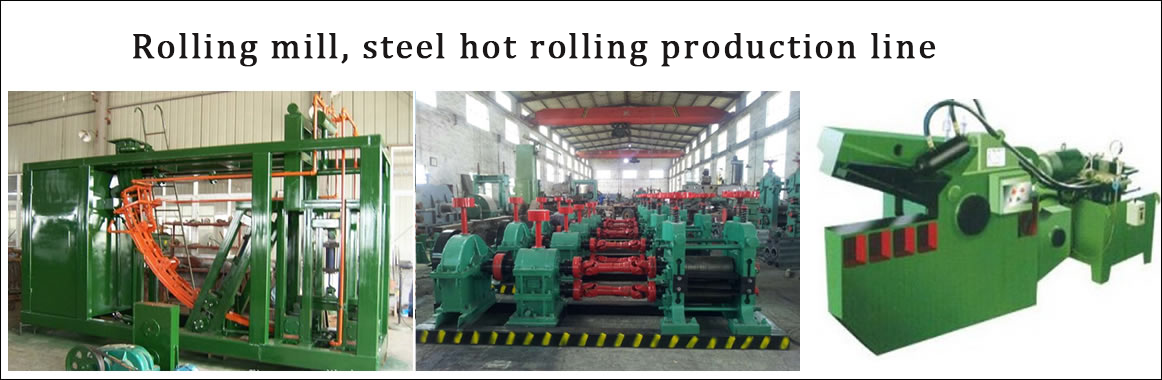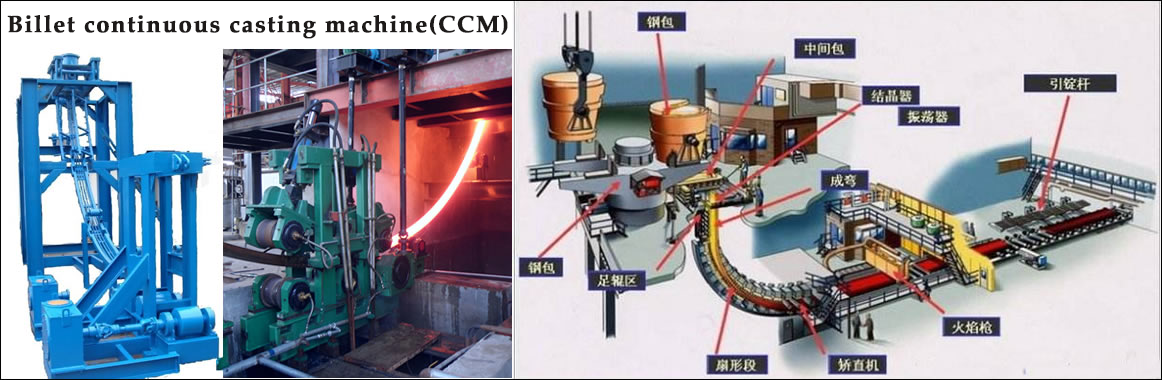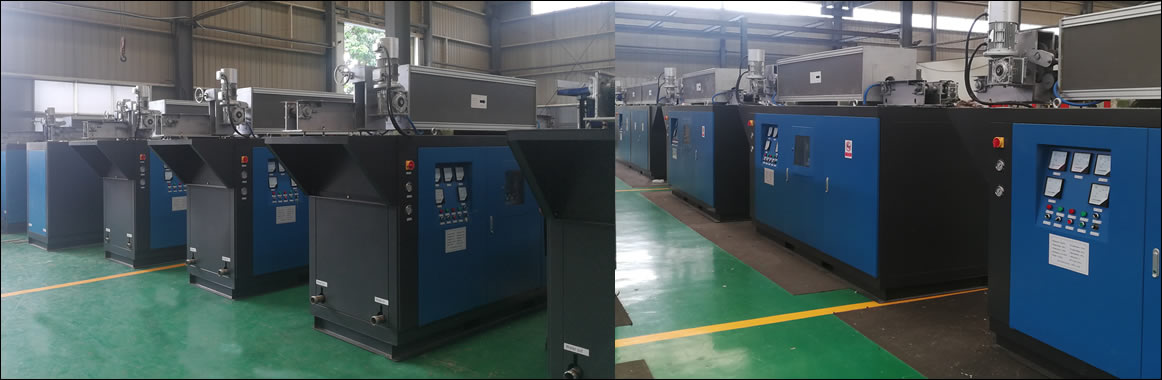••••••Contact
Tel: 18037961302
Sales hot line ( 24 hours  service): 18037961302

E-Mail: firstfurnace@gmail.com

whatsapp:+8618037961302

Adress: Luoxin Industrial Park, Luoyang, Henan
Electric furnace principle

Research on Harmonic Detection and Reactive Compensation Technology of 3T Melting Furnace

Overview of Harmonic Contamination Test for Nonlinear Load of 3 Ton Melting Furnace

In order to find out the harmonic pollution of the nonlinear load of the 3 ton melting furnace , and provide the basis for harmonic control and reactive power compensation, we conducted harmonic testing on site. The system supplies power to the 10kV distribution line. The main relevant parameters of the distribution transformer matched with the intermediate frequency electric furnace are as follows : the model is ZS 2 500/10 , the rated voltage is 10kV±5%/ 9 50V , the rated current of 10kV side is 86.6A , short circuit impedance. 6.15% . The harmonic current generated during the process of refining a furnace steel was tested.

Harmonic pollution test for non-linear load of 3 tons of melting furnace : National Standard GB/T 14549-93 "Power Quality Harmonics of Public Grid":

3 tons melting furnace nonlinear load harmonic pollution field test results and analysis of test time at 20:45 on May 8th, 2009

Test point : the low voltage outlet side of the transformer, the main circuit breaker outlet end of the switchgear.

Below there is 1# switchgear measurement data

Harmonic pollution test conclusion of nonlinear load of 3 tons melting furnace

According to the above data analysis, there is a large reactive power, and the total current distortion rate (THD) on the low-voltage side of the transformer is about 28% , which exceeds the national standard. The harmonic current injected into the grid during the operation of the intermediate frequency electric furnace exceeds the standard. Therefore, measures to suppress harmonics can be taken locally at the harmonic source.

Harmonic pollution control scheme for nonlinear load of 3 tons melting furnace

According to the analysis of the field data, there is a large reactive power. Therefore, from the perspective of cost-effective optimization, it is proposed to use a hybrid filter to compensate the reactive power and harmonics in the field. The structure is shown inFigure 4.1 :

In the above scheme, the passive filter is mainly used to filter out the 5th and 7th harmonics with higher content , and is used to compensate the reactive power, while the active filter is used to filter out the high-order fractional harmonics. To suppress the effect of resonance.

Assuming the compensated target power factor cosφ2=0.96 , then :

Fundamental reactive power QC=βav Pc (tanφ1 -tanφ2)

Where : Pc― maximum active computing load (kW) :

Avav - average load rate, generally can be taken from 0.7 to 0.8 :

Φ1― the power factor angle before compensation, cosφ1=0.86 :

Φ2― the power factor angle after compensation, cosφ2=0.96 :

Take the data in Table 3.1 and calculate that QC = 296 kvar ( three-phase )

The passive filter is designed as two sets of single-tuned wave filters with a design capacity of 330 kvar ( three-phase ) for compensating for reactive and 5th and 7th harmonics.

The active filter is designed to shunt active power filter, the capacity is determined by the SA = 3EICh

Where E- voltage RMS

ICh - harmonic current RMS

Take the data in Table 3.2 and calculate that SA = 86.4kVA ( three-phase ) , use 100kVA to suppress the elimination of 11-25 harmonics.

According to the data, the capacity of the filter is selected. The characteristics of the above scheme are as follows: the parallel compensation scheme is adopted, and the system is connected into the grid in parallel as a whole. The hardware circuit is simple and convenient for on-site installation: centralized compensation of the transformer outlet end, using a hybrid filtering scheme The main subharmonic is filtered by the passive filter, which reduces the capacity of the active filter and reduces the system cost: the active control part compensates the current in real time to follow the change of its command current signal, which is closed loop control.

Harmonic pollution results of nonlinear load of 3 tons of melting furnace

Based on the introduction of harmonic control principle, this paper analyzes the structural characteristics of various filters. Through the detection of 3T melting furnace widely used in China , the scheme of harmonic control and reactive power compensation is proposed. The use of a hybrid filter not only effectively suppresses harmonics, but also provides sufficient compensation for reactive power. At the same time, the energy saving effect is expected to reach more than 10% . As China's power quality requirements continue to increase, hybrid filters have huge market potential.

Research on Harmonic Detection and Reactive Compensation Technology of 3T Melting Furnace